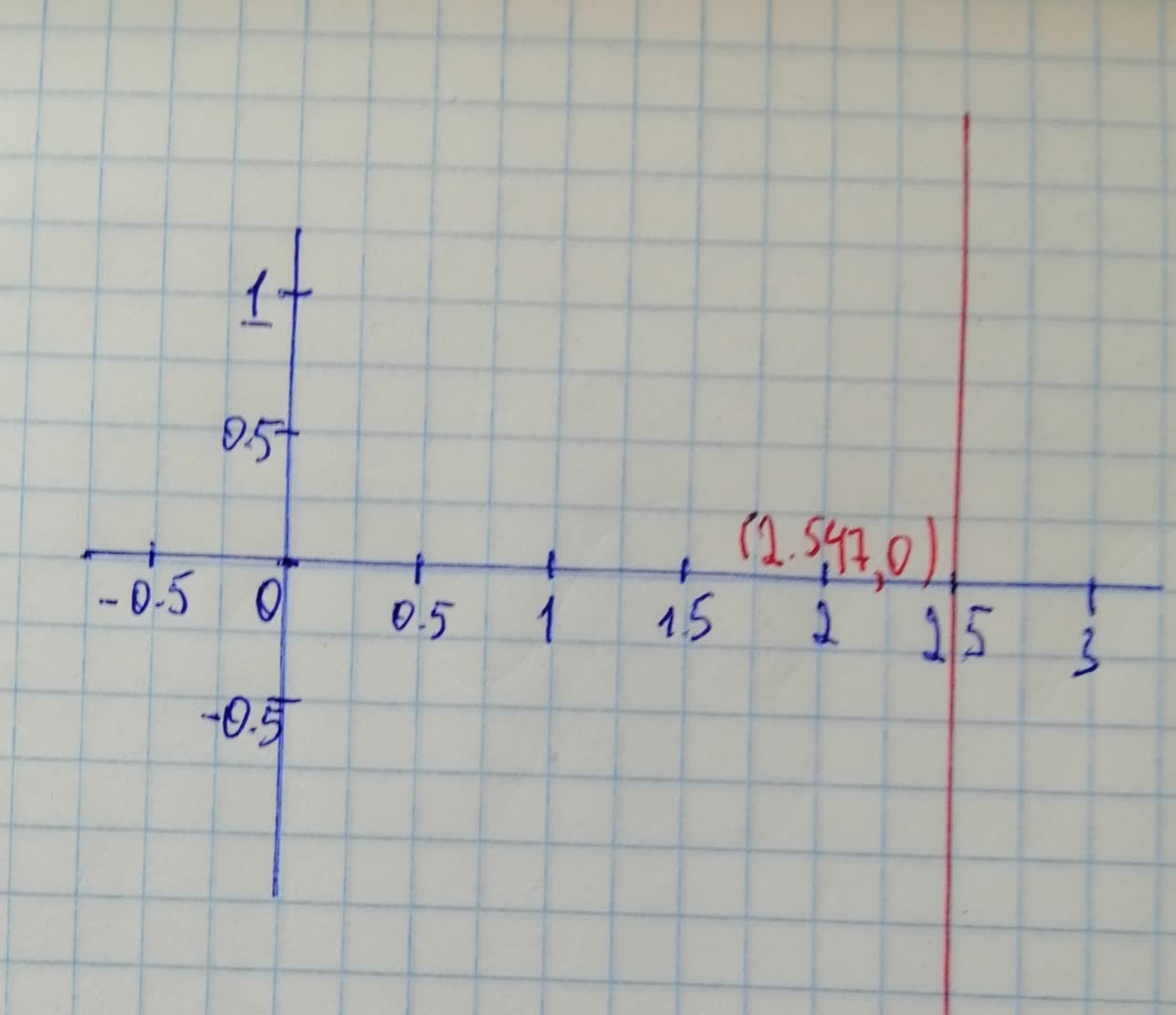Question# Solving Equations: graphically find all real solutions of the equation x^{3}-2x^{2}-x-1=0

Decimals
ANSWEREDSolving Equations Graphically Find all real solutions of the equation, rounded to two decimals.
$$\displaystyle{x}^{{{3}}}-{2}{x}^{{{2}}}-{x}-{1}={0}$$2021-08-04
Step 1
Given,
$$\displaystyle{x}^{{{3}}}-{2}{x}^{{{2}}}-{x}-{1}={0}$$
To solve any equation graphically, draw the graph of the given equation and find out the point at which it cuts the x-axis.Step 2
Hence, from the graph, it is quite clear that the equation has only 1 real solution, i.e. $$\displaystyle{x}={2.547}$$. Rounding off to 2 decimals, $$\displaystyle{x}={2.55}$$ is the only real solution for $$\displaystyle{x}^{{{3}}}-{2}{x}^{{{2}}}-{x}-{1}={0}$$